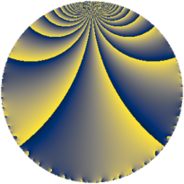# Properties

 Label 600.2.a.hLevel $600$ Weight $2$ Character orbit 600.a Self dual yes Analytic conductor $4.791$ Analytic rank $0$ Dimension $1$ CM no Inner twists $1$

# Related objects

## Newspace parameters

 Level: $$N$$ $$=$$ $$600 = 2^{3} \cdot 3 \cdot 5^{2}$$ Weight: $$k$$ $$=$$ $$2$$ Character orbit: $$[\chi]$$ $$=$$ 600.a (trivial)

## Newform invariants

 Self dual: yes Analytic conductor: $$4.79102412128$$ Analytic rank: $$0$$ Dimension: $$1$$ Coefficient field: $$\mathbb{Q}$$ Coefficient ring: $$\mathbb{Z}$$ Coefficient ring index: $$1$$ Twist minimal: no (minimal twist has level 24) Fricke sign: $$-1$$ Sato-Tate group: $\mathrm{SU}(2)$

## $q$-expansion

 $$f(q)$$ $$=$$ $$q + q^{3} + q^{9} + O(q^{10})$$ $$q + q^{3} + q^{9} + 4q^{11} + 2q^{13} - 2q^{17} - 4q^{19} + 8q^{23} + q^{27} + 6q^{29} + 8q^{31} + 4q^{33} - 6q^{37} + 2q^{39} - 6q^{41} - 4q^{43} - 7q^{49} - 2q^{51} + 2q^{53} - 4q^{57} + 4q^{59} - 2q^{61} + 4q^{67} + 8q^{69} + 8q^{71} - 10q^{73} - 8q^{79} + q^{81} + 4q^{83} + 6q^{87} - 6q^{89} + 8q^{93} - 2q^{97} + 4q^{99} + O(q^{100})$$

## Embeddings

For each embedding $$\iota_m$$ of the coefficient field, the values $$\iota_m(a_n)$$ are shown below.

For more information on an embedded modular form you can click on its label.

Label $$\iota_m(\nu)$$ $$a_{2}$$ $$a_{3}$$ $$a_{4}$$ $$a_{5}$$ $$a_{6}$$ $$a_{7}$$ $$a_{8}$$ $$a_{9}$$ $$a_{10}$$
1.1
 0
0 1.00000 0 0 0 0 0 1.00000 0
 $$n$$: e.g. 2-40 or 990-1000 Significant digits: Format: Complex embeddings Normalized embeddings Satake parameters Satake angles

## Atkin-Lehner signs

$$p$$ Sign
$$2$$ $$1$$
$$3$$ $$-1$$
$$5$$ $$1$$

## Inner twists

This newform does not admit any (nontrivial) inner twists.

## Twists

By twisting character orbit
Char Parity Ord Mult Type Twist Min Dim
1.a even 1 1 trivial 600.2.a.h 1
3.b odd 2 1 1800.2.a.m 1
4.b odd 2 1 1200.2.a.d 1
5.b even 2 1 24.2.a.a 1
5.c odd 4 2 600.2.f.e 2
8.b even 2 1 4800.2.a.q 1
8.d odd 2 1 4800.2.a.cc 1
12.b even 2 1 3600.2.a.v 1
15.d odd 2 1 72.2.a.a 1
15.e even 4 2 1800.2.f.c 2
20.d odd 2 1 48.2.a.a 1
20.e even 4 2 1200.2.f.b 2
35.c odd 2 1 1176.2.a.i 1
35.i odd 6 2 1176.2.q.a 2
35.j even 6 2 1176.2.q.i 2
40.e odd 2 1 192.2.a.b 1
40.f even 2 1 192.2.a.d 1
40.i odd 4 2 4800.2.f.d 2
40.k even 4 2 4800.2.f.bg 2
45.h odd 6 2 648.2.i.b 2
45.j even 6 2 648.2.i.g 2
55.d odd 2 1 2904.2.a.c 1
60.h even 2 1 144.2.a.b 1
60.l odd 4 2 3600.2.f.r 2
65.d even 2 1 4056.2.a.i 1
65.g odd 4 2 4056.2.c.e 2
80.k odd 4 2 768.2.d.d 2
80.q even 4 2 768.2.d.e 2
85.c even 2 1 6936.2.a.p 1
95.d odd 2 1 8664.2.a.j 1
105.g even 2 1 3528.2.a.d 1
105.o odd 6 2 3528.2.s.j 2
105.p even 6 2 3528.2.s.y 2
120.i odd 2 1 576.2.a.d 1
120.m even 2 1 576.2.a.b 1
140.c even 2 1 2352.2.a.i 1
140.p odd 6 2 2352.2.q.l 2
140.s even 6 2 2352.2.q.r 2
165.d even 2 1 8712.2.a.u 1
180.n even 6 2 1296.2.i.e 2
180.p odd 6 2 1296.2.i.m 2
220.g even 2 1 5808.2.a.s 1
240.t even 4 2 2304.2.d.k 2
240.bm odd 4 2 2304.2.d.i 2
260.g odd 2 1 8112.2.a.be 1
280.c odd 2 1 9408.2.a.h 1
280.n even 2 1 9408.2.a.cc 1
420.o odd 2 1 7056.2.a.q 1

By twisted newform orbit
Twist Min Dim Char Parity Ord Mult Type
24.2.a.a 1 5.b even 2 1
48.2.a.a 1 20.d odd 2 1
72.2.a.a 1 15.d odd 2 1
144.2.a.b 1 60.h even 2 1
192.2.a.b 1 40.e odd 2 1
192.2.a.d 1 40.f even 2 1
576.2.a.b 1 120.m even 2 1
576.2.a.d 1 120.i odd 2 1
600.2.a.h 1 1.a even 1 1 trivial
600.2.f.e 2 5.c odd 4 2
648.2.i.b 2 45.h odd 6 2
648.2.i.g 2 45.j even 6 2
768.2.d.d 2 80.k odd 4 2
768.2.d.e 2 80.q even 4 2
1176.2.a.i 1 35.c odd 2 1
1176.2.q.a 2 35.i odd 6 2
1176.2.q.i 2 35.j even 6 2
1200.2.a.d 1 4.b odd 2 1
1200.2.f.b 2 20.e even 4 2
1296.2.i.e 2 180.n even 6 2
1296.2.i.m 2 180.p odd 6 2
1800.2.a.m 1 3.b odd 2 1
1800.2.f.c 2 15.e even 4 2
2304.2.d.i 2 240.bm odd 4 2
2304.2.d.k 2 240.t even 4 2
2352.2.a.i 1 140.c even 2 1
2352.2.q.l 2 140.p odd 6 2
2352.2.q.r 2 140.s even 6 2
2904.2.a.c 1 55.d odd 2 1
3528.2.a.d 1 105.g even 2 1
3528.2.s.j 2 105.o odd 6 2
3528.2.s.y 2 105.p even 6 2
3600.2.a.v 1 12.b even 2 1
3600.2.f.r 2 60.l odd 4 2
4056.2.a.i 1 65.d even 2 1
4056.2.c.e 2 65.g odd 4 2
4800.2.a.q 1 8.b even 2 1
4800.2.a.cc 1 8.d odd 2 1
4800.2.f.d 2 40.i odd 4 2
4800.2.f.bg 2 40.k even 4 2
5808.2.a.s 1 220.g even 2 1
6936.2.a.p 1 85.c even 2 1
7056.2.a.q 1 420.o odd 2 1
8112.2.a.be 1 260.g odd 2 1
8664.2.a.j 1 95.d odd 2 1
8712.2.a.u 1 165.d even 2 1
9408.2.a.h 1 280.c odd 2 1
9408.2.a.cc 1 280.n even 2 1

## Hecke kernels

This newform subspace can be constructed as the intersection of the kernels of the following linear operators acting on $$S_{2}^{\mathrm{new}}(\Gamma_0(600))$$:

 $$T_{7}$$ $$T_{11} - 4$$

## Hecke characteristic polynomials

$p$ $F_p(T)$
$2$ $$T$$
$3$ $$-1 + T$$
$5$ $$T$$
$7$ $$T$$
$11$ $$-4 + T$$
$13$ $$-2 + T$$
$17$ $$2 + T$$
$19$ $$4 + T$$
$23$ $$-8 + T$$
$29$ $$-6 + T$$
$31$ $$-8 + T$$
$37$ $$6 + T$$
$41$ $$6 + T$$
$43$ $$4 + T$$
$47$ $$T$$
$53$ $$-2 + T$$
$59$ $$-4 + T$$
$61$ $$2 + T$$
$67$ $$-4 + T$$
$71$ $$-8 + T$$
$73$ $$10 + T$$
$79$ $$8 + T$$
$83$ $$-4 + T$$
$89$ $$6 + T$$
$97$ $$2 + T$$